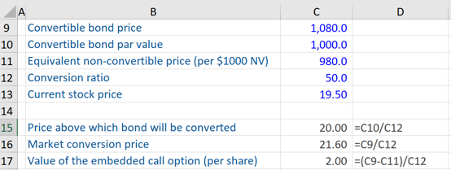## What are “Convertible Securities”?

Convertible securities are essentially an asset or investment which can be transformed or ‘converted’ into another asset type. This is usually within a ‘qualifying period’ in terms of time and price. An example is a ‘convertible bond’ – a bond which can also be converted into equity (assuming certain criteria are met to make this financially attractive). A convertible bond will likely pay a lower coupon rate than a regular bond, but it does offer the potential upside of converting into equity if certain conditions are met.

More specifically, convertible bonds may be converted into a predetermined quantity of the issuer’s equity, typically at the discretion of the bondholder. The benefit this gives to the investor is that at predetermined dates should they choose to do so, they can convert the debt into equity i.e. shares – benefiting from the good performance of the company’s stock price.

In terms of the conversion of debt into equity, the bond indenture will specify this conversion rate – giving the number of shares of common stock that may be issued per bond. This is in effect a core provision that could benefit the investor. This benefit also allows the issuing company to reduce their borrowing costs as it will reduce the amount of interest that they otherwise would have to pay.

## Key Learning Points

• Convertible securities allow investors to buy an asset that can be later ‘converted’ into another type of asset
• If the appropriate criteria in terms of timing and price are met, investors can achieve additional upside from the asset switch on top of the fixed amount usually paid from the convertible security
• With reference to the conversion of debt into equity, there is a market conversion price
• Other kinds of convertible products include Warrants and Convertible Preferred Stock
• The outcome of converting debt into equity on the calculation of Earnings per Share (EPS) is unknown due to the changes in no shares and debt repayments

## Market Conversion Price, Warrants, Convertible Preferred Stock and EPS

When looking at convertible securities, it is important to understand the potential upside or downside of converting what is usually a bond into equity. The market conversion price is the effective cost of buying shares through the convertible bond.

The formula is:

Market conversion price = Convertible bond price / Conversion ratio

There are several types of convertible products including warrants and convertible preferred stock.

Warrants:  these are typically issued with a bond and the warrant will allow the holder to purchase a predetermined number of shares in the issuing company at a fixed (exercise) price. Warrants are economically identical to a convertible bond, but the bond and warrant can be traded separately.

When a warrant is issued with a bond, it is very similar to a convertible bond. At the end of the life of the bond, you can take the redemption proceeds as cash, or you convert that into a fixed number of shares.

Convertible Preferred Stock: this is preferred stock (that pays a fixed dividend) but the investor can decide to convert into a predetermined number of shares of the common stock of the issuer.

An important point worth stating is that converting debt to equity increases the issuer’s number of shares outstanding. Further, in terms of the impact on a company’s earnings per share (EPS), if the debt is converted into equity it reduces the amount of interest to be paid – boosting the company’s earnings, but it also increases the number of shares in issue, and the increase in the denominator in the earnings per share (EPS) calculation. Therefore, the outcome on earnings per share (EPS) calculation of a company’s convertible debt being converted into shares is typically hard to determine.

## Convertible Bond, Example

Given below is an example of a convertible bond. Here we have calculated the stock price at which the bond will be converted, the market conversion price (which is the effective price of buying shares through the convertible bond) and the value of the call option embedded in the convertible bond on a per-share basis.The convertible bond price is \$1,080 and its par value is \$1,000. We also have the price of an equivalent non-convertible bond of \$980. We are told the conversion ratio is 50. This effectively means we can get 50 shares per \$1,000 of par value should we choose to convert on the current underlying stock price of shares – which is  \$19.50. Now the price at which the bond will be converted at the end of the life of the convertible bond is the convertible bond’s par value divided by the conversion ratio. This calculation yields \$20.

So, if the stock price is above \$20, it makes sense to take the 50 shares that will be worth more than \$1,000. However, if the stock price is below \$20, the 50 shares that you could convert the bond into will be less than \$1,000, so it won’t be sensible to convert them into shares. Investors should just take the redemption cash flow on the bond of \$1,000.

Next, the market conversion price is calculated as the price of the convertible bond divided by the conversion ratio i.e. it is \$21.60. If an investor is buying a convertible bond to access the shares, then the investor is effectively paying \$21.60 per share.

Lastly, the value of the call option embedded within the convertible bond can be identified by looking at the difference in price between the convertible bond and the equivalent non-convertible bond. If we take this difference of \$100 and divide it by the conversion ratio of 50 i.e. we obtain a value of \$2.00 per share for the value of the call option.